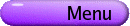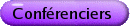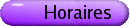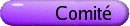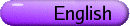Probabilité
Org: Martin Barlow et Edwin Pekins (UBC)
[PDF]

Morita approximations to random copolymer localization
[PDF]

We look at Morita approximations to analyze Dyck path models of random copolymer localization at an interface between two immiscible liquids. A polymer can be either delocalized, with zero density of monomers at the interface, or localized, with a non-zero density of monomers at the interface.

The phase diagram for the first order Morita approximation, which constrains the number of one type of comonomers, consists of four distinct regions: two delocalized regions, a localized region, and a region of coexistence of the two delocalized regions.

It is known that the boundaries of the delocalized regions do not change as the order of the Morita approximation is increased. It is also known that the region of coexistence of the two delocalized regions is not present in the quenched model, it is a localized region instead. We have some numerical evidence that points toward the existence of the region of coexistence of the two delocalized regions for Morita approximations of up to eighth order. We also have some arguments that point toward localization in the region of coexistence of the two delocalized regions, for Morita approximations of twelfth order and above.

We get tighter upper bounds on the average constrained free energy as the order of the Morita approximation increases.

OMER ANGEL, UBC
1 Dimensional DLA
[PDF]

Diffusion limited aggregation (DLA) in 2 or more dimensions is an infamously difficult model for the growth of a random fractal. DLA was introduced in 1981 and attracted massive attention (63,400 Google results for "diffusion limited aggregation"). Kesten's 1987 upper bound is almost the only proven result on it.

We define a variation of DLA in one dimension. This becomes interesting when the random walk generating the DLA has arbitrary long jumps. It turns out that the growth rate of the aggregate depends on the step distribution in complex ways. In particular there are three phase transitions in the behaviour when the step distribution has finite expectation, finite variance, and finite third moment.

Joint work with Gidi Amir, Itai Benjamini and Gadi Kozma.

BALINT VIRAG, University of Toronto
Noise limits for complex eigenvalues
[PDF]

Consider a Coulomb gas in the plane: n points distributed with density proportional to product |xi-xj|2 with respect to some underlying measure m.

When m is Gaussian, this process coincides with the eigenvalues of matrix filled with i.i.d. complex normal entries. Other choices of m yield the zeros of i.i.d. Gaussian power series, the projection of the spherical gas, or the eigenvalues of a random unitary matrix.

When the points get denser, these processes converge to noises that are different from the usual white one.

This is joint work with Brian Rider.

DAVID BRYDGES, University of British Columbia
Joint density for the local times of continuous-time random walks
[PDF]

We investigate the local times of continuous-time random walks on arbitrary discrete state spaces. For fixed finite range of the random walk, we derive an explicit formula for the joint density of all local times on the range, at any fixed time. We apply this formula in the following directions:

(1) we prove the analog of the well-known Ray-Knight description of Brownian local times for continuous-time simple random walk on Z,
(2) we derive large deviation estimates for exponential integrals of the normalized local times beyond the exponential scale.

This work was done in collaboration with Remco van der Hofstad and Wolfgang Koenig.

JEAN-FRANCOIS DELMAS, ENPC, 6-8 av. Blaise Pascal, 77455 Champs sur Marne, France
Fragmentation associated to continuous random trees
[PDF]

This is a joint work with R. Abraham. There is a natural way to consider continuous limit of Galton-Watson trees, using Lévy process with no negative jumps (see the work of T. Duquesne, J.-F. Le Gall and Y. Le Jan). Each node of the continuous random tree has a mass corresponding to the "number" of its children. We cut each node at independent exponential random time with parameter equal to the mass of the node. This procedure gives a collection of smaller and smaller sub-trees. Considering the evolution of the sequence of the sizes of the subtrees as time goes on, we get a fragmentation process. In the stable case, this corresponds to the self-similar fragmentation described by G. Miermont. In the general case, we get a non self-similar fragmentation process. We compute for the general fragmentation process a family of dislocation measures as well as the law of the size of a tagged fragment.

ANTAL JARAI, Carleton University, Ottawa, ON
A self-organized critical forest fire model
[PDF]

We study a simple forest fire model introduced by physicists Drossel and Schwabl. In a certain limiting case, the model is expected to behave similarly to critical statistical physics systems. Extensive simulation results are available in the literature, but hardly anything seems to be known rigorously, especially in dimensions two and higher. We analyze the one-dimensional model, which already shows quite non-trivial behaviour. We are able to give bounds on the cluster size distribution that suggest that this distribution shows interesting behaviour on two separate scales. For "small" clusters we expect a power law, and for "large" clusters another non-trivial distribution.

Joint work with Jacob van den Berg.

RICHARD KENYON, UBC, Vancouver, BC
Groves, Pfaffians, and crossing probabilities
[PDF]

A planar essential spanning forest is a spanning forest of a planar graph in which every component touches the outer boundary. The components of such a spanning forest define a partition of the boundary vertices. Given a random planar essential spanning forest of a finite planar graph with n boundary vertices, we show how to compute the probability of any particular partition as a rational function of the pairwise resistances.

Groves, defined by Carroll and Speyer, are certain kinds of essential spanning forests on planar graphs which arose in their study of the "cube recurrence relation". We show that a certain class of topologies of essential spanning forests, including groves, can be computed using Pfaffians. The proof is a generalization of the Karlin-McGregor-Lindstrom-Gessel-Viennot method.

This is joint work with David Wilson.

MIKE KOZDRON, University of Regina
Loop-erased random walk and Fomin's identity
[PDF]

In this talk, I will outline the recent proof of a 2001 conjecture of S. Fomin concerning a relationship between crossing probabilities of two-dimensional loop-erased random walk and planar Brownian motion. This conjecture was proved by establishing estimates of random walk exit probabilities in simply connected domains. These estimates can also be used to give a quick derivation of a crossing exponent for loop-erased random walk first proved by R. Kenyon in 2000.

This talk is based on joint work with Greg Lawler.

VLADA LIMIC, University of British Columbia, Vancouver, BC V6T 1Z2
Recent progress on the attracting edge problem
[PDF]

Reinforcement is observed frequently in nature and society, where beneficial interactions tend to be repeated. An edge reinforced random walker on a graph remembers the number of times each edge was traversed in the past, and decides to make the next random step with probabilities favouring places visited before. Using martingale techniques and comparison with the generalized Urn scheme, it can be shown that the edge reinforced random walker on a graph of bounded degree, with the reinforcement weight function W(k) = kr, r > 1, traverses a random attracting edge at all large times, with probability 1. A remarkably short argument of Sellke (1994) shows that attracting edge exists if and only if

 å k 1 W(k) < ¥,
whenever the underlying graph has no odd cycle. The conjecture that the above summability condition implies existence of attracting edge when the underlying graph is a triangle is still open. Progress has been made recently towards better understanding of attracting edge property for a large class of weights W satisfying the above condition.

JEREMY QUASTEL, University of Toronto
Travelling fronts in the stochastically perturbed KPP equation
[PDF]

We study the asymptotic speed of travelling fronts in a reaction-diffusion equation perturbed by a Fisher-Wright white noise. We prove part of a conjecture of Brunet and Derrida that such perturbations have an unusually strong effect on the front speed.

This is joint work with Carl Mueller (Rochester) and Leonid Mytnik (Technion).

BRUCE REED, McGill University, Montreal
On the diameter of a random Minimum Weight Spanning Tree
[PDF]

In first passage percolation, given random weights on the edges of a connected graph G, we are interested in the structure of the graph formed by the union of the lightest paths from some source s to every other vertex. A natural variant would be to consider the lightest graph containing a path between every pair of vertices (as we will see this makes sense even in the infinite setting). These objects arise naturally in computer science and network design and are called Minimum Weight Spanning Trees.

In such a tree, there is a unique path between every pair of vertices. We are interested in the maximum number of edges in such a path, which is known as the diameter of the tree. We consider weighting the edges of a complete graph (the mean field model) with i.i.d. weights, conditioning on the weights being distinct (a probability 1 event if our distribution has no point mass).

Amongst other results on its distribution, we show that for any weighting, the expected value of the diameter for the MWST on a complete graph with n vertices is between c1 n[ 1/3] and c2n[ 1/3] for two constants c1 and c2.

This is joint work with Louigi Addario-Berry at end.

TOM SALISBURY, York University and the Fields Institute
Singular stochastic integral equations
[PDF]

I will describe work in progress concerning Yamada-Watanabe type singular solutions to stochastic integral equations. Complete results in this direction would establish pathwise uniqueness for SPDE models analogous to catalytic super Brownian motion. Unfortunately we don't have complete results, but I will try to describe what progress has been made to date.

This is joint work with Leonid Mytnik (Technion).